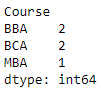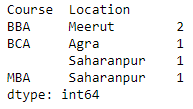Related Articles

# How to count duplicates in Pandas Dataframe?

• Last Updated : 28 Jul, 2020

Let us see how to count duplicates in a Pandas DataFrame. Our task is to count the number of duplicate entries in a single column and multiple columns.

Under a single column : We will be using the `pivot_table()` function to count the duplicates in a single column. The column in which the duplicates are to be found will be passed as the value of the `index` parameter. The value of `aggfunc `will be ‘size’.

 `# importing the module``import` `pandas as pd``  ` `# creating the DataFrame``df ``=` `pd.DataFrame({``'Name'` `: [``'Mukul'``, ``'Rohan'``, ``'Mayank'``, ``                             ``'Sundar'``, ``'Aakash'``],``                   ``'Course'` `: [``'BCA'``, ``'BBA'``, ``'BCA'``, ``'MBA'``, ``'BBA'``],``                   ``'Location'` `: [``'Saharanpur'``, ``'Meerut'``, ``'Agra'``, ``                                 ``'Saharanpur'``, ``'Meerut'``]})`` ` `# counting the duplicates``dups ``=` `df.pivot_table(index ``=` `[``'Course'``], aggfunc ``=``'size'``)`` ` `# displaying the duplicate Series``print``(dups)`

Output :Across multiple columns : We will be using the `pivot_table()` function to count the duplicates across multiple columns. The columns in which the duplicates are to be found will be passed as the value of the `index` parameter as a list. The value of `aggfunc `will be ‘size’.

 `# importing the module``import` `pandas as pd``  ` `# creating the DataFrame``df ``=` `pd.DataFrame({``'Name'` `: [``'Mukul'``, ``'Rohan'``, ``'Mayank'``, ``                             ``'Sundar'``, ``'Aakash'``],``                   ``'Course'` `: [``'BCA'``, ``'BBA'``, ``'BCA'``, ``'MBA'``, ``'BBA'``],``                   ``'Location'` `: [``'Saharanpur'``, ``'Meerut'``, ``'Agra'``, ``                                 ``'Saharanpur'``, ``'Meerut'``]})`` ` `# counting the duplicates``dups ``=` `df.pivot_table(index ``=` `[``'Course'``, ``'Location'``], aggfunc ``=``'size'``)`` ` `# displaying the duplicate Series``print``(dups)`

OutputAttention geek! Strengthen your foundations with the Python Programming Foundation Course and learn the basics.

To begin with, your interview preparations Enhance your Data Structures concepts with the Python DS Course. And to begin with your Machine Learning Journey, join the Machine Learning – Basic Level Course

My Personal Notes arrow_drop_up